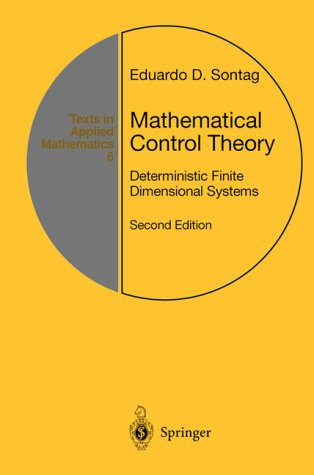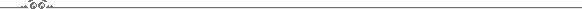# Mathematical Control TheoryNow online version available (click on link for pdf file, 544 pages)

(Please note: book is copyrighted by Springer-Verlag. Springer has kindly allowed me to place a copy on the web, as a reference and for ease of web searches. Please consider buying your own hardcopy.)Precise reference:
Eduardo D. Sontag, Mathematical Control Theory: Deterministic Finite Dimensional Systems.
Second Edition
, Springer, New York, 1998.
(531+xvi pages, ISBN 0-387-984895)
Series: Textbooks in Applied Mathematics, Number 6. Hardcover, approx \$55.00
Order in USA from 1-800-SPRINGER or from amazon.com.Errata for 2nd editionFirst Edition's web page.
(Errata, revisions, and some comments, all regarding the first edition, are included there. No errata posted since mid 1997.)Math ReviewsPublicity Blurb:

This textbook introduces the core concepts and results of Control and System Theory. Unique in its emphasis on foundational aspects, it takes a "hybrid" approach in which basic results are derived for discrete and continuous time scales, and discrete and continuous state variables. Primarily geared towards mathematically advanced undergraduate or graduate students, it may also be suitable for a second engineering course in control which goes beyond the classical frequency domain and state-space material. The choice of topics, together with detailed end-of-chapter links to the bibliography, makes it an excellent research reference as well.

The Second Edition constitutes a substantial revision and extension of the First Edition, mainly adding or expanding upon advanced material, including: Lie-algebraic accessibility theory, feedback linearization, controllability of neural networks, reachability under input constraints, topics in nonlinear feedback design (such as backstepping, damping, control-Lyapunov functions, and topological obstructions to stabilization), and introductions to the calculus of variations, the maximum principle, numerical optimal control, and linear time-optimal control.

Also covered, as in the First Edition, are notions of systems and automata theory, and the algebraic theory of linear systems, including controllability, observability, feedback equivalence, and minimality; stability via Lyapunov, as well as input/output methods; linear-quadratic optimal control; observers and dynamic feedback; Kalman filtering via deterministic optimal observation; parametrization of stabilizing controllers, and facts about frequency domain such as the Nyquist criterion.

From the reviews of the first edition:

"This book will be very useful for mathematics and engineering students interested in a modern and rigorous systems course, as well as for experts in control theory and applications"
Mathematical Reviews
"An excellent book... gives a thorough and mathematically rigorous treatment of control and system theory"
Zentralblatt fur Mathematik
"The style is mathematically precise... fills an important niche... serves as an excellent bridge (to topics treated in traditional engineering courses). The book succeeds in conveying the important basic ideas of mathematical control theory, with appropriate level and style"
IEEE Transactions on Automatic ControlIntroduction
What Is Mathematical Control Theory?
Proportional-Derivative Control
Digital Control
Feedback Versus Precomputed Control
State-Space and Spectrum Assignment
Outputs and Dynamic Feedback
Dealing with Nonlinearity
A Brief Historical Background
Some Topics Not Covered Systems
Basic Definitions
I/O Behaviors
Discrete-Time
Linear Discrete-Time Systems
Smooth Discrete-Time Systems
Continuous-Time
Linear Continuous-Time Systems
Linearizations Compute Differentials
More on Differentiability
Sampling
Volterra Expansions
Reachability and Controllability
Basic Reachability Notions
Time-Invariant Systems
Controllable Pairs of Matrices
Controllability Under Sampling
More on Linear Controllability
Bounded Controls
First-Order Local Controllability
Controllability of Recurrent Nets
Piecewise Constant Controls
Nonlinear Controllability
Lie Brackets
Lie Algebras and Flows
Accessibility Rank Condition
Necessity of Accessibility Rank Condition
Feedback and Stabilization
Constant Linear Feedback
Feedback Equivalence
Feedback Linearization
Disturbance Rejection and Invariance
Stability and Other Asymptotic Notions
Unstable and Stable Modes
Lyapunov and Control-Lyapunov Functions
Linearization Principle for Stability
Introduction to Nonlinear Stabilization
Outputs
Basic Observability Notions
Time-Invariant Systems
Continuous-Time Linear Systems
Linearization Principle for Observability
Realization Theory for Linear Systems
Recursion and Partial Realization
Rationality and Realizability
Abstract Realization Theory
Observers and Dynamic Feedback
Observers and Detectability
Dynamic Feedback
External Stability for Linear Systems
Frequency-Domain Considerations
Parametrization of Stabilizers
Optimality: Value Function
Dynamic Programming
Tracking and Kalman Filtering
Nonlinear Stabilizing Optimal Controls
Optimality: Multipliers
Review of Smooth Dependence
Unconstrained Controls
Excursion into the Calculus of Variations
Constrained Controls: Minimum Principle
Optimality: Minimum-Time for Linear Systems
Existence Results
Maximum Principle for Time-Optimality
Applications of the Maximum Principle
Remarks on the Maximum Principle
Appendix: Linear Algebra
Operator Norms
Singular Values
Jordan Forms and Matrix Functions
Continuity of Eigenvalues
Appendix: Differentials
Finite Dimensional Mappings
Maps Between Normed Spaces
Appendix: Ordinary Differential Equations
Review of Lebesgue Measure Theory
Initial-Value Problems
Existence and Uniqueness Theorem Linear Differential Equations
Stability of Linear Equations
Bibliography
List of Symbols
IndexBack to Eduardo Sontag's Public Homepage.